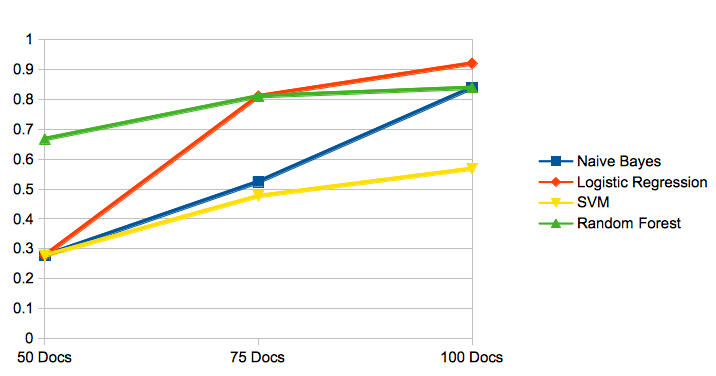# Steps

1. Data loading and tagging — parse data using segmentation on Khmer text
2. Extract features from the document using TFIDF
3. Run a few different ML algorithms and compare the results
4. Save the chosen model and load it to run it in production

`select id, title, body, category from dbo.article WHERE category IS NOT NULL`
`(docIds, doc_titles, doc_contents, categories) = getArticles();`
`របស់យើងប្រសើរជាងមុន។ => របស់ យើង ប្រសើរ ជាង មុន។`

## 2. Extracting Features

`from sklearn.feature_extraction.text import TfidfVectorizer`
`def tokenizersplit(str):    return str.split();tfidf = TfidfVectorizer(tokenizer=tokenizersplit, encoding='utf-8', min_df=2, ngram_range=(1, 2), max_features=25000)tfidf_vect.fit(df['text'])tfidf_vect.transform(df['text'])`
• encoding: is set to ‘utf-8’ to handle Khmer Unicode characters
• min_df: ignore term with number doc count less than a given value. Value 2 means, a term must exist at least on 2 documents to be counted.
• ngram_range: ngram you want to extract (more detail below)
• max_features: max number of features
`Naive Bayes accuracy:         0.63, 0.63Logistic Regression accuracy: 0.90, 0.96SVM accuracy:                 0.50, 0.50Random Forest accuracy:       0.83, 0.96`
`Naive Bayes accuracy:         0.60, 0.60Logistic Regression accuracy: 0.77, 0.80SVM accuracy:                 0.50, 0.50Random Forest accuracy:       0.83, 0.93`

## 3. Evaluate Performance with Different ClassifierAccuracy on different algorithms on 3 different training size

## Performance analysis

`from sklearn import model_selection, linear_model, metrics# split the dataset into training and validation datasetstrain_x, valid_x, train_y, valid_y = model_selection.train_test_split(df['text'], df['cat'], test_size=0.35, random_state=0)from sklearn import metricsm=linear_model.LogisticRegression()m.fit(xtrain_tfidf, train_y)y_pred = m.predict(xvalid_tfidf)print(metrics.classification_report(valid_y, y_pred, '''## 85 docs for training, 15 docs for validation set             precision    recall  f1-score   supportno_accident       0.93      1.00      0.96        13   accident       1.00      0.92      0.96        13avg / total       0.96      0.96      0.96        26## 65 docs on training, 35 docs for validation set             precision    recall  f1-score   supportno_accident       0.80      1.00      0.89        20   accident       1.00      0.71      0.83        17avg / total       0.89      0.86      0.86        37'''`

## 4. Save Model and Load it Run in Production

`import pickle;tfidf = TfidfVectorizer(tokenizer=tokenizersplit, encoding=’utf-8');tfidf.fit(df.text);pickle.dump(tfidf, open(‘feature_100.pkl’, ‘wb’));`
`...model = linear_model.LogisticRegression()model.fit(features, labels)import picklepickle.dump(model, open("model.pkl", 'wb'))`
`import pickle# needed to load pickle tfidfdef tokenizersplit(str):    return str.split();tfidf = pickle.load(open('feature_100.pkl', 'rb'))...loaded_model = pickle.load(open('model.pkl', 'rb'))`

# Steps by Steps detail on Training Process

1. Load training documents
`(docIds, docTitles, docBodies, categories) = getArticles();`
`(token_bodies, token_titles) = tokenizeDocs(docTitles, docBodies);# concatenate title, body with space into tokenTexttokenText = [token_titles[i] + " " + token_bodies[i] for i in xrange(len(token_titles))]import pandas as pddf = pd.DataFrame({id: docIds});df[‘text’] = tokenText;df[‘cat’] = categories;`
`tfidf = TfidfVectorizer(tokenizer=tokenizersplit, encoding=’utf-8');tfidf.fit(df.text);`
`import pickle;pickle.dump(tfidf, open(‘feature_100.pkl’, ‘wb’));`
`features = tfidf.transform(df.text)`
`from sklearn import model_selection, preprocessing# split the dataset into training and validation datasetstrain_x, valid_x, train_y, valid_y = model_selection.train_test_split(df['text'], df['cat'], test_size=0.30, random_state=1)# label encode the target variableencoder = preprocessing.LabelEncoder()train_y = encoder.fit_transform(train_y)valid_y = encoder.fit_transform(valid_y)`
`xtrain_tfidf =  tfidf_vect.transform(train_x)xvalid_tfidf =  tfidf_vect.transform(valid_x)`
`from sklearn import metrics, linear_model, naive_bayes, metrics, svm, xgboostdef train_model(classifier, trains, t_labels, valids, v_labels):    # fit the training dataset on the classifier    classifier.fit(trains, t_labels)    # predict the labels on validation dataset    predictions = classifier.predict(valids)    return metrics.accuracy_score(predictions, v_labels)# Naive Bayesaccuracy = train_model(naive_bayes.MultinomialNB(), xtrain_tfidf, train_y, xvalid_tfidf, valid_y);print "NB accuracy: ", accuracy; # 94%, 65%, 60%, 60%# Logistic Regressionaccuracy = train_model(linear_model.LogisticRegression(), xtrain_tfidf, train_y, xvalid_tfidf, valid_y);print "LR accuracy: ", accuracy; # 96%, 84%, 94%, 100%, 97%# SVMaccuracy = train_model(svm.SVC(), xtrain_tfidf, train_y, xvalid_tfidf, valid_y);print "SVM accuracy: ", accuracy; # 54%, 48%, 48%# Random Forestaccuracy = train_model(ensemble.RandomForestClassifier(), xtrain_tfidf, train_y, xvalid_tfidf, valid_y)print "RF accuracy: ", accuracy # 94% ,97%, 94%, 85%# Extereme Gradient Boosting (not from scikit-learn)accuracy = train_model(xgboost.XGBClassifier(), xtrain_tfidf.tocsc(), train_y, xvalid_tfidf.tocsc());print "Xgb accuracy: ", accuracy;  # 82%, 91%,92%`
`# convert cat ("accident/non-accident) into category_id 0,1df['category_id'] = df['cat'].factorize(sort=True)labels = df.category_idfeatures = tfidf.transform(df.text)model = linear_model.LogisticRegression()model.fit(features, labels)import picklepickle.dump(model, open("model.pkl", 'wb'))`

# Steps by Steps to Run in Production

`(all_documents, doctitles, docIds) = getNewArticles()`
`(tokenized_documents, tokenized_document_title) = tokenizeDocs(all_documents, doctitles);# concatenate title, body with space into tokenTexttokenText = [doctitles[i] + " " + all_documents[i] for i in xrange(len(doctitles))]import pandas as pddf = pd.DataFrame({id: docIds});df[‘text’] = tokenText;`
`# needed to load pickle feature_100.pkldef tokenizersplit(str):    return str.split();# load tfidf.fittfidf = pickle.load(open('feature_100.pkl', 'rb'))features = tfidf.transform(df.text)`
`features = tfidf.transform(df.text)`
`import pickleloaded_model = pickle.load(open('model.pkl', 'rb'))y_pred = loaded_model.predict(features)`
`df['tag'] = y_predprint(df[[id,'tag']])`

# Conclusion

`# 20% validation setNB accuracy:  0.960784313725LR accuracy:  0.941176470588SVM accuracy:  0.666666666667RF accuracy:  0.911764705882Xgb accuracy:  0.980392156863# 25% validation setLR accuracy:  0.934210526316SVM accuracy:  0.684210526316RF accuracy:  0.960526315789Xgb accuracy:  0.986842105263# 30% validation setNB accuracy:  0.947368421053LR accuracy:  0.940789473684SVM accuracy:  0.644736842105RF accuracy:  0.953947368421Xgb accuracy:  0.960526315789`